×

Search anything:

# Basic Guide to Python Itertools and its functionalities

#### Software Engineering PythonFREE BOOK -> Problems for the day before your Coding Interview (on Amazon)

The Python Itertools module is a standard library module provided by Python 3 Library that provide various functions to work on iterators to create fast , efficient and complex iterations.

In this article , I will explain each function starting with a basic definition and a standard application of the function using a python code snippet and its output. But before we delve into the deeper aspects of the python Itertools module, let us understand what makes it stand out among others.

## How to get Itertools module?

Python Itertools is a standard module available in the Python 3 Library. We can import the module along with thw operator module using the following steps.

``````import itertools
import operator
``````

The operatoe module is not necessary for basic application of itertools , but is essential when facing advanced problems using multiple uses of Itertools iterators.

## What is Itertools and what makes it special?

As said previously,by definition,The Python Itertools module is a standard library module provided by Python 3 Library that provide various functions working together in combinations to work on making 'iterator algebra' faster and more efficient. This module uses a set of iterator building blocks inspired by constructs from APL , Haskell, and SML ; making them work efficiently for pure Python.

For Example ,you have two lists and want to multiply the element of both the lists together, there are various approaches to this problem. The standard approach would be to tediously iterate all the single element of both the lists and multiplying them. The other approach would be use the itertools module function Operator.mul as first parameter of the map function and the lists as the second and third respectively.

This utility of providing the developer of multiple ways to approach a particular problem using iterators make the itertools module more applicable to the developers and managers by reducing time and space complexities, and in turn , increasing efficiency.

There are Three different types of Iterators provided by this module:

1. Infinite Iterators
2. Combinatoric Iterators
3. Terminating Iterators

## Infinite Iterators

Infinite iterators are such kind of iterators in which the iterator object do not exhaust, or is infinite. Python has in-built iterators such as lists , tuples and dictionaries. But it is not necessary that an iterator object should exhaust, which become infinite iterators.

This module has three infinite iterators:

1. count()
2. cycle()
3. repeat()
Iterator Arguments Example
count() start, step count(10) --> 10 11 12 13 14 ...
cycle() a cycle('ABCD') --> A B C D A B C D ...
repeat() val, num repeat(10, 3) --> 10 10 10

### count()

This iterator prints evenly spaced numbers staring from the number start indefinitely . Normally , If steps are mentioned, then the number skipped is 1 by default.

#### Code

``````import itertools
for i in itertools.count(5, 1):
if i == 15:
break
else:
print(i, end =" ")
``````

#### Output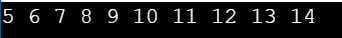### cycle()

This iterator prints all the elements in a cyclic fashion from the container. When the iterable is exhausted it, returns to the beginning and starts printing from the start indefinitely.

#### Code

``````import itertools
count = 0
for i in itertools.cycle('123'):
if count > 7:
break
else:
print(i, end = " ")
count += 1
``````

#### Output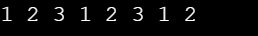### repeat()

This iterator results in the object being printed infinitely. if the optional keyword num is mentioned in the program , then the object gets printed the mentioned number of times. This iterator is often used with zip() to create an invariant part of a tuple record.

#### Code

``````import itertools
print ("Printing numbers repeatedly : ")
print (list(itertools.repeat(100, 4)))
``````

#### Output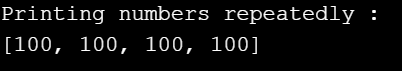## Combinatoric Iterators

Combinatoric iterators are recursive iterators which are used for simplifying combinational constructs such as permutations, combinations and and cartesian products.
There are four combinatoric iterators included in the module:-

1. product()
2. permutations()
3. combinations()
4. combinations_with_replacements()
Iterator Arguments Example
product() p,q,r,... product('1234', repeat=2)
permutations() p,r permutations('1234', 2)
combination() p,r combinations('1234', 2)
combinations_with_replacements() p,r combinations_with_replacement('1234', 2)

### product()

This iteration computes the cartesian product of two input iterables. The output are stored in tuples in sorted form , to specify the number of repetitions , the keyword repeat is used.

#### Code

``````from itertools import product
print("The cartesian product of the containers:")
print(list(product('AB', [3, 4])))
``````

#### Output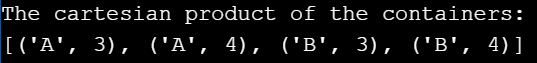### permutations()

This generates all the possible permutations of an iterable and returns r length permutations of the elements of the iterable. If r is not specified , then r defaults to the length of the iterable.Elements are treated as unique based on their position, not on their value. So if the input elements are unique, there will be no repeat values in each permutation.

#### Code

``````from itertools import permutations
print ("All the permutations of the given string is:")
print (list(permutations('12')))
print()
print ("All the permutations of the given container is:")
print(list(permutations(range(3), 2)))
``````

#### Output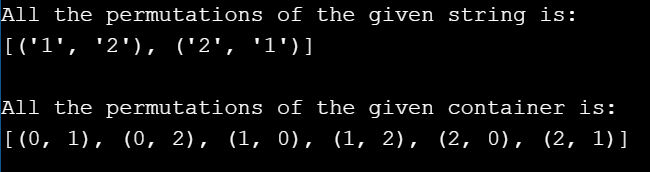### combinations()

This iterator returns all possible combinations without repetition of the iterables and r length subsequences of elements from the input iterable.If the input iterable is sorted, the combination tuples will be produced in sorted order.Elements are treated as unique based on their position, not on their value. So if the input elements are unique, there will be no repeat values in each permutation.

#### Code

``````from itertools import combinations
print ("All the combination of string in sorted order(without replacement) is:")
print(list(combinations('AB', 2)))
print()
``````

#### Output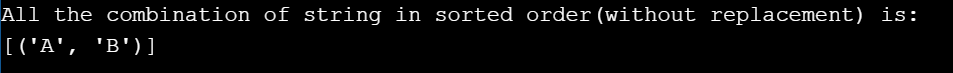### combinations_with_replacement()

This iterator returns all possible combinations with repetition of the iterables and r length subsequences of elements from the input iterable, So , there can be multiple outputs with same iterable but different positions.If the input iterable is sorted, the combination tuples will be produced in sorted order.Elements are treated as unique based on their position, not on their value. So if the input elements are unique, there will be no repeat values in each permutation.

#### Code

``````from itertools import combinations_with_replacement
print ("All the combination of string in sorted order(with replacement) is:")
print(list(combinations_with_replacement("AB", 2)))
print()
``````

#### Output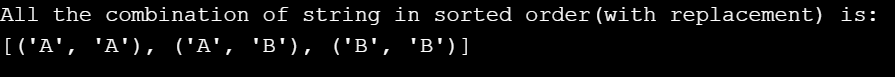## Terminating Iterators

Terminating iterators produce the output based on the functionality of the the method used . These mostly work on short input sequences.

Different types of iterators along with their arguments and examples are given in the table below:-

Iterator Arguments Example
accumulate() iterable ,function accumulate([1,2,3,4,5]) --> 1 3 6 10 15
chain() iterable1 ,iterable2, ... chain('ABC', 'DEF') --> A B C D E F
chain.from_iterable() iterable chain.from_iterable(['ABC', 'DEF']) --> A B C D E F
compress() iterable ,selector compress('ABCDEF', [1,0,1,0,1,1]) --> A C E F
dropwhile() function ,sequence dropwhile(lambda x: x<5, [1,4,6,4,1]) --> 6 4 1
filterfalse() function, sequence filterfalse(lambda x: x%2, range(10)) --> 0 2 4 6 8
groupby() iterable ,key key_func = lambda x: x itertools.groupby(L, key_func):
islice() iterable, start, stop, step islice('ABCDEFG', 2, None) --> C D E F G
starmap() function ,sequence starmap(pow, [(2,5), (3,2), (10,3)]) --> 32 9 1000
takewhile() func ,iterable takewhile(lambda x: x<5, [1,4,6,4,1]) --> 1 4
tee() iterable ,count it = itertools.tee(iti, 3) iti = [2, 4, 6, 7, 8, 10, 20]
zip_longest() iterable1 ,iterable2 zip_longest('ABCD', 'xy', fillvalue='-') --> Ax By C- D-

### accumulate()

The iterator returns the sums or the accumulated results of other binary functions of the iterables.
Then , the iterator returns the sums or the accumulated results of other binary functions of the iterables.If no Function is defined ,then addition is the default function. The output depends on the input iterable. so if the input is empty, output will be too.

#### Code

``````import itertools
import operator
li1 = [1, 4, 5, 7]
print ("The product after each iteration is : ", end ="")
print (list(itertools.accumulate(li1, operator.mul)))
``````

#### Output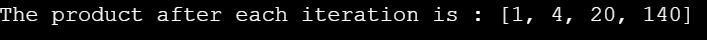### chain()

This iterator returns all the elements of the first iterable until it is exhausted and continues to the next till it is exhausted.

#### Code

``````import itertools
li1 = [1, 4, 5, 7]
li2 = [1, 6, 5, 9]
print ("All values in mentioned chain are : ", end ="")
print (list(itertools.chain(li1, li2)))
``````

#### Output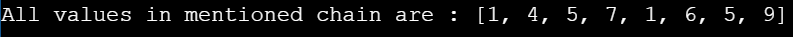### chain.from_iterable()

Alternate iterator to chain() but the argument here is any iterable container such as a list.

#### Code

``````import itertools
li1 = [1, 4, 5, 7]
li2 = [1, 6, 5, 9]
li3 = [8, 10, 5, 4]
li4 = [li1, li2, li3]
print ("All values in mentioned chain are : ", end ="")
print (list(itertools.chain.from_iterable(li4)))
``````

#### Output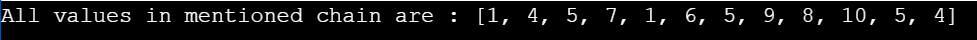### compress()

The iterators returns the elements from the container in which the boolean value returns as true according to the boolean value list passed as other arguments. If no element is true according to the list all are skipped and the iterator returns a null value.

#### Code

``````import itertools
print ("The compressed values in string are : ", end ="")
print (list(itertools.compress('ILOVESOCCER', [1, 0, 0, 0, 0, 1, 0, 0, 1, 0, 0, 0, 0])))
print (list(itertools.compress('ILOVESOCCER', [0, 0, 0, 0, 0, 0, 0, 0, 0, 0, 0, 0, 0])))
``````

#### Output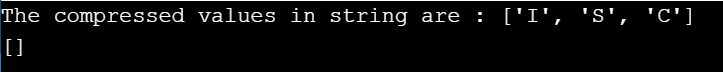### dropwhile()

The iterator drops elements till the predicate is true and after the first instance of false, it returns every element till the iterable is exhausted.

#### Code

``````import itertools
li = [2, 4, 5, 7, 8]
print ("The values after condition returns false : ", end ="")
print (list(itertools.dropwhile(lambda x : x % 2 == 0, li)))
``````

#### Output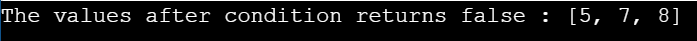### filterfalse()

As per the name , the iterator only returns elements with False predicates. If the predicate is none, then returns all elements as false.

#### Code

``````import itertools
li = [2, 4, 5, 7, 8 ,10 ,1 , 12 , 14 ,13 ]
print ("The values that return false to function are : ", end ="")
print (list(itertools.filterfalse(lambda x : x % 2 == 0, li)))
``````

#### Output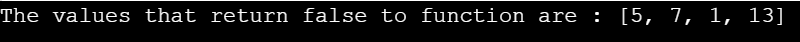### groupby()

This iterator returns keys and iterables of grouped items after calculating each element consecutively.The key is a function computing a key value for each element. If not specified or is None, key defaults to an identity function and returns the element unchanged.

#### Code

``````import itertools
a_list = [("Animal", "cat"),
("Animal", "dog"),
("Bird", "peacock"),
("Bird", "pigeon")]
an_iterator = itertools.groupby(a_list, lambda x : x)
for key, group in an_iterator:
key_and_group = {key : list(group)}
print(key_and_group)
``````

#### Output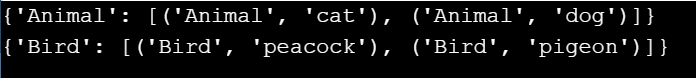### islice()

The iterator returns specified elements from the iterable. islice() does not support negative values for start and stop, unlike regular splicing. If the start is non-zero , it skips elements till it returns the first element. If stop is not mentioned , then the elements are returned till the iterable is exhausted.

#### Code

``````import itertools
li = [2, 4, 5, 7, 8, 10, 20]
print ("The sliced list values are : ", end ="")
print (list(itertools.islice(li, 1, 7, 1)))
print ("The sliced list values are : ", end ="")
print (list(itertools.islice(li, 1, 6, 2)))
``````

#### Output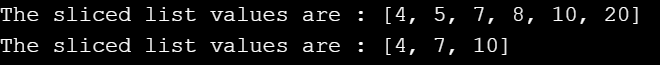### starmap()

The iterator takes a function and a tuple list as arguments and computes the function using arguments from the iterable. Basically , starmap() considers each element of an iterable within another iterable as a separate item.

The main difference between the Built-in function map() and starmap() is that map() considers each tuple within the list as a single argument and generates an error when it gets in-sufficient arguments which starmap() overcomes. It can also be used in practical aspects such as if one wants to different functions to different elements of the list rather than using map() for each element.

#### Code

``````from itertools import starmap
co_ordinates =[(2, 3, 4),
(3, 4, 5),
(6, 8, 10),
(1, 5, 7),
(7, 4, 10)]
right_triangles = list(starmap(lambda x, y, z:True
if ((x * x)+(y * y))==(z * z)
else False, co_ordinates))
print("tuples which form right angled triangle :",
right_triangles, end ="\n")
print("The right triangle coordinates are :",
end ="")
for i in range (0, len(right_triangles)):
if right_triangles[i]== True:
print(co_ordinates[i], end =" ")
``````

#### Output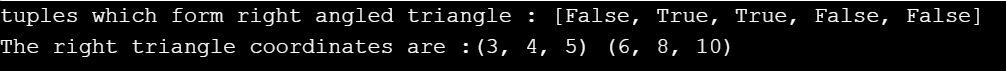### takewhile()

The iterator returns elements till the predicate is true. Once the predicate turns false for the first time the iterator drops all elements from the iterable till the iterable is exhausted. It is directly opposite in functionality to dropwhile().

#### Code

``````import itertools
li = [2, 4, 6, 7, 8, 10, 20]
print ("The list values till 1st false value are : ", end ="")
print (list(itertools.takewhile(lambda x : x % 2 == 0, li )))
``````

#### Output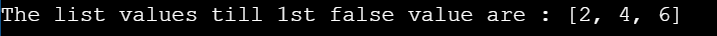### tee()

The iterator divides the input iterable into n numner of independant iterables mentioned in the argument. tee() sorts the sequence in FIFO(FIRST IN FIRST OUT) sequence , so the first element of the input iterable gets first output in the independant output iterables.

#### Code

``````import itertools
li = [2, 4, 6, 7, 8, 10, 20]
iti = iter(li)
it = itertools.tee(iti, 3)
print ("The iterators are : ")
for i in range (0, 3):
print (list(it[i]))
``````

#### Output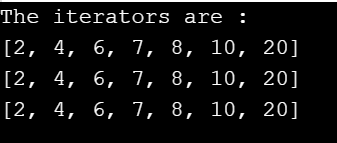### zip_longest()

The iterator aggregates the elements from both the iterables. This continues till the longest iterable is exhausted. If both iterables have uneven lenghths , the missing values are filled with fillvalue().

#### Code

``````from itertools import zip_longest
x =[1, 2, 3, 4, 5, 6, 7]
y =[8, 9, 10]
z = list(zip_longest(x, y))
print(z)
``````

#### Output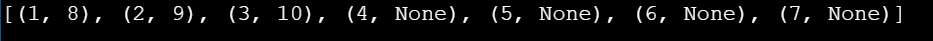I hope that with this article at OpenGenus, I could shed some light on the topic of different functionalities of Itertools . itertools is a powerful module in the Python standard library, and an essential tool to have in your toolkit. With it, you can write faster and more memory efficient code that is often simpler and easier to read. There are many more applications using multiple uses of itertools which can be discussed. If anything, though, itertools is a testament to the power of iterators and lazy evaluation. Even though there are many examples in this article , this is just the tip of the iceberg that is Itertools.

I hope you all good luck and keep exploring.